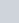Huma, Hubna and Seema received a total of ₹ 2,016
Question:

Huma, Hubna and Seema received a total of ₹ 2,016 as monthly allowance from their mother such that Seema gets ½ of what Huma gets and Hubna getstimes Seema’s share. How much money do the three sisters get individually?

Solution:

From the question it is given that,

Total monthly allowance received by Huma, Hubna and Seem = ₹ 2,016

from their mother

Seema gets allowance = ½ of Huma’s share

Hubna gets allowance =of Seema’s share

= 5/3 of Seema’s share

= 5/3 of ½ of Huma’s share … [∵ given]

= 5/3 × ½ of Huma’s share

= 5/6 of Huma’s share

So,

Huma’s share + Hubna’s share + Seema’s share = ₹ 2,016

Let Huma’s share be 1,

1 + (5/6) Huma’s share + ½ Huma’s share = ₹ 2,016

(1 + (5/6) + ½) = ₹ 2,016

The LCM of the denominators 1, 6 and 2 is 6

(1/1) = [(1×6)/ (1×6)] = (6/6)

(5/6) = [(5×1)/ (6×1)] = (5/6)

(1/2) = [(1×3)/ (2×3)] = (3/6)

Then,

(6/6) + (5/6) + (3/6) = ₹ 2,016

(6 + 5 + 3)/ 6 = ₹ 2,016

(14/6) = ₹ 2,016

So, Huma’s share = ₹ 2,016 ÷ (14/6)

= 2,016 × (6/14)

= 144 × 6

∴Huma’s Share is = ₹ 864

Seema’s share = ½ Huma’s share

= ½ × 864

= ₹ 432

Hubna’s share = 5/6 of Huma’s share

= 5/6 × 864

= 5 × 144

= ₹ 720